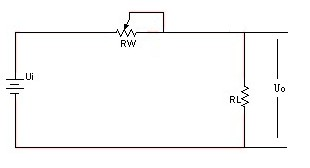Home >> ARTICLES >>
Linear Voltage Regulator DC Power Supply Is Characterized
Basic introduction
According to the adjustment of pipe work, we often regulated power supply is divided into two categories: linear regulated power supply and switch regulated power supply. In addition, there is a use of the regulator of the small power source. The linear regulated power supply, means for adjusting the transistor operating in the linear state under the DC regulated power supply. Adjusting the transistor operating in the linear state, so to understand: RW (see the following analysis ) is a continuous variable, which is linear. In the switching power supply is not the same, the switch tube ( in switch power supply, we see the adjusting tube called the switch tube ) is working in the open, closed under two different conditions: Open -- resistance is small; off -- high resistance. Work in the switch state of pipe is not linear state. Linear regulated power supply is relatively early in the use of a class of DC regulated power supply
.Linear regulated power supply

Power characteristic
Linear voltage regulator DC power supply is characterizedby : the output voltage is lower than an input voltage; reaction speed, small output ripple; work to produce low noise; low efficiency ( now often see LDO is to solve efficiency problem appears); heat ( especially large power supply ), indirectly to increase system thermal noise.

Working principle
We first use the chart to illustrate the principle of linear regulated power supply voltage regulation. As shown in the figure, a variable resistor RW and a load resistor RL to form a voltage divider circuit, the output voltage is:Uo =" Ui" RL / ( RW+RL ), so by adjusting the RW of the size, you can change the output voltage. Please note that, in this way, if we only look at the adjustable resistor RW value changes, the output of the Uo is not linear, but if RW and RL together, it is linear. Also note that this map, we will not RW terminal painted even to the left, and the painting on the right. Although this view from the formulas and no difference, but the picture on the right side, it reflects the" sampling" and" feedback" concept ---- actual power, mostly working in sampling and feedback mode, using feedforward method rarely, or is used, also is only an auxiliary method.
Let us continue: if we use a three transistor or field effect tube, instead of drawing the rheostat, and by detecting the output voltage, to control the" value of the rheostat", that the output voltage remains constant, so we can achieve the goal of stabilizing voltage. The three transistor or FET is used to adjust the output voltage size, so called the adjusting tube.Linear regulated power supply

As shown in the figure that, because the adjustment tube in series with the power and load, it is called a stabilized power supply in series. Accordingly, there are parallel type regulated power supply, is to adjust tube with load parallel to regulate the output voltage, typical of the reference voltage stabilizer TL431 is a shunt regulator. The so-called parallel mean, like in Figure 2voltage regulator tube, through the shunt to ensure attenuation amplifier tube emitter voltage" stable", perhaps this map does not let you go see it as " parallel", but with a closer look, indeed. However, we also note here: the voltage regulator tube, is the use of its nonlinear area works, therefore, if you think it is a power, it is also a nonlinear power. In order to facilitate understanding, so we find a suitable diagrams, until you can simply read so far.
Because the adjusting tube is equal to a resistance, current flows through the resistor will heat, so the work in the linear condition adjustment tube, the general would produce large quantity of heat, causes the efficiency is not high. This is a linear regulated power supply is one of the most important one drawback. Want to know more details about linear regulated power supply, please refer to the textbook of analog electronic circuit. Here we mainly is to help you clarify these concepts and the relationship between them.
In general, linear regulated power supply by an adjusting tube, a reference voltage, a sampling circuit, an error amplifying circuit and so on several basic components. May also include some such as protection circuit, starting circuit and other parts. Below is a simple linear regulated power supply schematic (sketch, omitting the filter capacitors and other components ), a sampling resistor by sampling the output voltage, and is compared with a reference voltage, a comparison result by the error amplifier, control the adjusting tube conduction degree, so that the output voltage is stable.